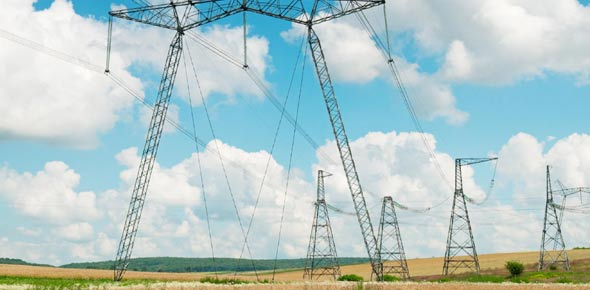# Electricity And Electronics Vocabulary Test! Trivia Quiz

10 Questions | Total Attempts: 1008SettingsElectricity can be defined as the flow of electric charge. Do you really know how electricity is generated and from where does it come? Take this vocabulary test and test your knowledge about basic vocabulary which covers questions from Electricity and Electronics. Read the questions carefully and answer. So, let's try out the quiz. All the best!

Related Topics
• 1.
___________________ is the electric pressure that causes current to flow.
• A.

Voltage

• B.

Watt

• C.

Ampere

• D.

Ohms

• E.

Joule

• 2.
__________________ is the opposition to the flow of electrons.
• A.

Resistance

• B.

Electricity

• C.

Conduction

• D.

Energy

• E.

Circuit

• 3.
___________________ is the flow of electrons in a wire or other conductor.
• A.

Current

• B.

Tides

• C.

Energy

• D.

Light

• E.

Waves

• 4.
A ___________________ is a device that changes mechanical energy into electrical energy.
• A.

Generator

• B.

Battery

• C.

Transformer

• D.

Motor

• E.

Charger

• 5.
A ______________ circuit contains components that are connected one after another.
• A.

Series

• B.

Resistance

• C.

Electrical

• D.

Fuse

• E.

None

• 6.
The capacitor capacitance is measured thru a unit called
• A.

Ohm

• B.

• C.

Hertz

• D.

Watt

• E.

Option 5

• 7.
How do you call the electronic component that works by transferring electrical current from one coil (called primary) to another coil (called secondary)?
• A.

Resistor

• B.

Transistor

• C.

Transformer

• D.

Diode

• 8.
Regarding diodes, we CANNOT state that:
• A.

They allow electrical current to flow on only direction, blocking currents coming from the oposite direction.

• B.

Every diode has polarity.

• C.

The positive pole is called anode and the negative pole is called cathode.

• D.

They can only work as conductors.

• 9.
On the US power grid, the alternating voltage has a frequency of:
• A.

60 Hz

• B.

50 Hz

• C.

110 Hz

• D.

220 Hz

• 10.
______________ integrated circuits are used on analog circuits such as amplifiers, oscillators and voltage regulators
• A.

Linear

• B.

Parallel

• C.

Digital

• D.

Bidirectional